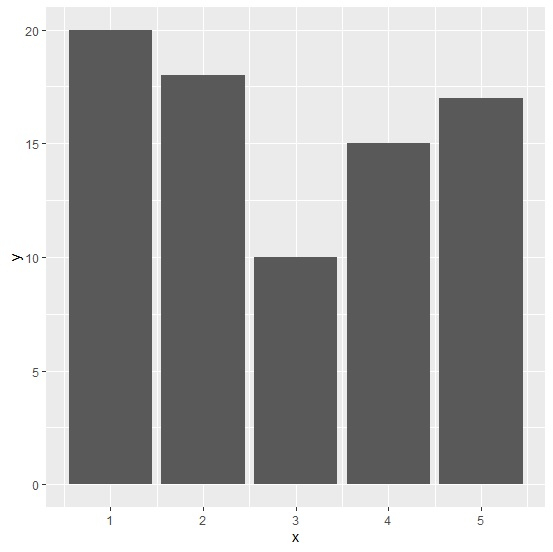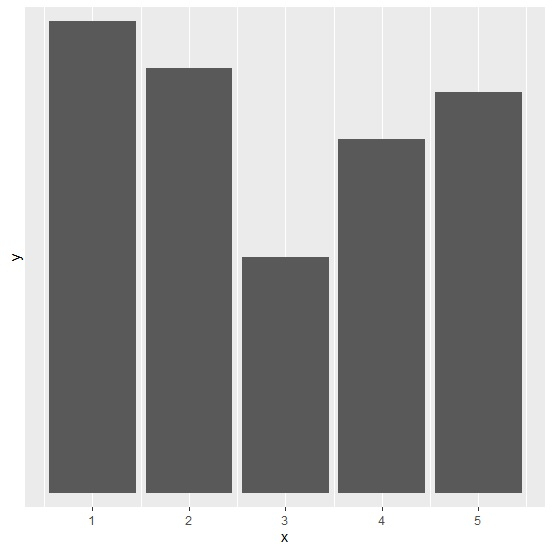# How to create a bar graph using ggplot2 without horizontal gridlines and Y-axes labels in R?

A bar graph plotted with ggplot function of ggplot2 shows horizontal and vertical gridlines. If we are interested only in the bar heights then we might prefer to remove the horizontal gridlines. In this way, we can have X-axis that helps us to look at the different categories we have in our variable of interest and get rid of the unnecessary information. This can be done by setting breaks argument to NULL in scale_y_discrete function.

## Example

Consider the below data frame −

> x<-1:5
> y<-c(20,18,10,15,17)
> df<-data.frame(x,y)

> library(ggplot2)

Creating the plot with all gridlines −

> ggplot(df,aes(x,y))+
+ geom_bar(stat='identity')

## OutputCreating the plot without horizontal gridlines −

> ggplot(df,aes(x,y))+
+ geom_bar(stat='identity')+
+ scale_y_discrete(breaks = NULL)

## Output